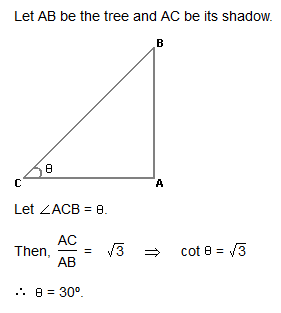Courses

# Test: Height And Distance

## 6 Questions MCQ Test Quantitative Aptitude (Quant) | Test: Height And Distance

Description
This mock test of Test: Height And Distance for CAT helps you for every CAT entrance exam. This contains 6 Multiple Choice Questions for CAT Test: Height And Distance (mcq) to study with solutions a complete question bank. The solved questions answers in this Test: Height And Distance quiz give you a good mix of easy questions and tough questions. CAT students definitely take this Test: Height And Distance exercise for a better result in the exam. You can find other Test: Height And Distance extra questions, long questions & short questions for CAT on EduRev as well by searching above.
QUESTION: 1

### Q. Two ships are sailing in the sea on the two sides of a lighthouse. The angle of elevation of the top of the lighthouse is observed from the ships are 30° and 45° respectively. If the lighthouse is 100 m high, the distance between the two ships is:

Solution:

Let AB be the lighthouse and C and D be the positions of the ships.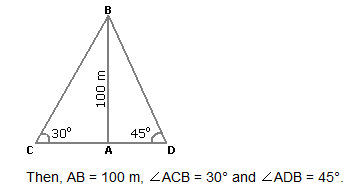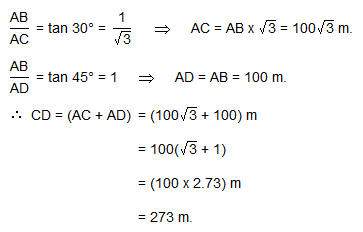QUESTION: 2

### A man standing at a point P is watching the top of a tower, which makes an angle of elevation of 30º with the man's eye. The man walks some distance towards the tower to watch its top and the angle of the elevation becomes 60º. What is the distance between the base of the tower and the point P?

Solution: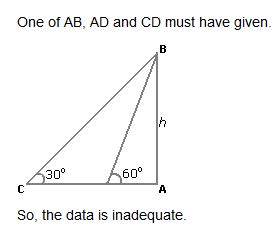QUESTION: 3

### The angle of elevation of a ladder leaning against a wall is 60º and the foot of the ladder is 4.6 m away from the wall. The length of the ladder is:

Solution: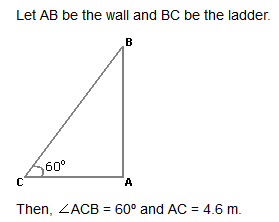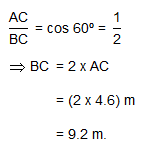QUESTION: 4

An observer 1.6 m tall is 20√3 away from a tower. The angle of elevation from his eye to the top of the tower is 30º. The heights of the tower is:

Solution: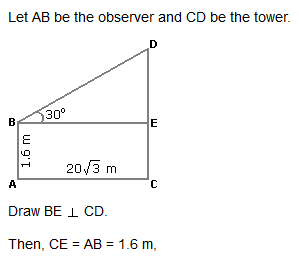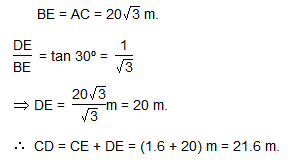QUESTION: 5

From a point P on a level ground, the angle of elevation of the top tower is 30º. If the tower is 100 m high, the distance of point P from the foot of the tower is:

Solution: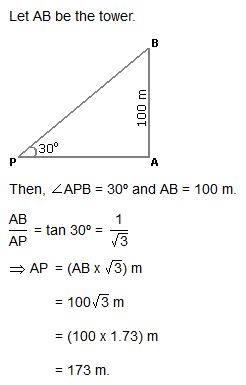QUESTION: 6

The angle of elevation of the sun, when the length of the shadow of a tree √3 times the height of the tree, is:

Solution: